## NCERT Solutions for Class 10 Maths Chapter 4 Quadratic Equations Ex 4.2

NCERT Solutions for Class 10 Maths Chapter 4 Quadratic Equations Ex 4.2 are part of NCERT Solutions for Class 10 Maths. Here we have given NCERT Solutions for Class 10 Maths Chapter 4 Quadratic Equations Ex 4.2.

 Board CBSE Textbook NCERT Class Class 10 Subject Maths Chapter Chapter 4 Chapter Name Quadratic Equations Exercise Ex 4.2 Number of Questions Solved 6 Category NCERT Solutions

## NCERT Solutions for Class 10 Maths Chapter 4 Quadratic Equations Ex 4.2

Ex 4.2 Class 10 NCERT Solutions Question 1.
Find the roots of the following quadratic equations by factorisation:
(i) x2 -3x – 10 = 0
(ii) 2x2 + x – 6 = 0
(iii) √2x2 + 7x + 5√2 = 0
(iv) 2x2 – x + $$\frac { 1 }{ 8 }$$ = 0 8
(v) 100 x2 – 20 X + 1 = 0
Solution: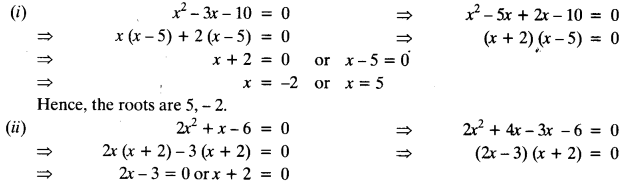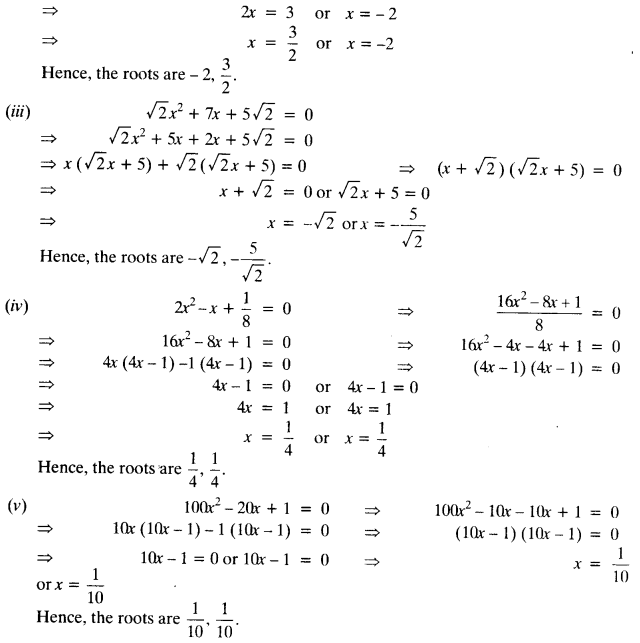Class 10 Maths 4.2 NCERT Solutions Question 2.
Solve the following situations mathematically:

(i) John and Jivanti together have 45 marbles. Both of them lost 5 marbles each and the product of the number of marbles they now have is 124. We would like to find out how many marbles they had to start with.
(ii) A cottage industry produces a certain number of toys in a day. The cost of production of each toy (in rupees) was found to be 55 minus the number of toys produced in a day. On a particular day, the total cost of production was ₹750. We would like to find out the number of toys produced on that day.
Solution:
(i) Let the number of marbles John had be x
Then, the number of marbles Jivanti had = 45 -x
The number of marbles left with John, when he lost 5 marbles = x – 5
The number of marbles left with Jivanti, when she lost 5 marbles = 45 -x – 5 = 40 -x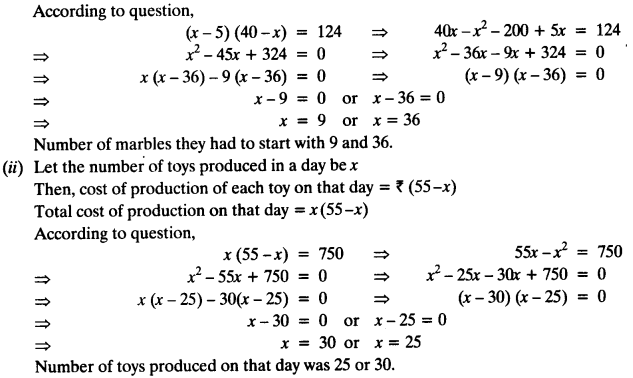Ex 4.2 Class 10 Question 3.
Find two numbers whose sum is 27 and product is 182.
Solution:
Let one number = x ,
∴ other number = 21 -x
According to question,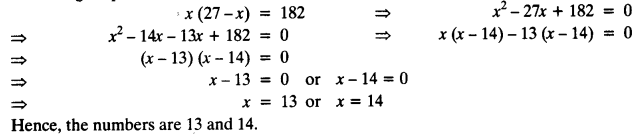Question 4.
Find two consecutive positive integers, sum of whose squares is 365.
Solution:
Let the two consecutive integers be x and x + 1.
According to question,Ex 4.2 Class 10 Question 5.
The altitude of a right triangle is 7 cm less than its base. If the hypotenuse is 13 cm, find the other two sides.
Solution:
Let base = x cm,
∴ height = (x – 7) cm
By Pythagoras Theorem,
(base)² + (height)² = (hypotenuse)²Ex 4.2 Class 10 Question 6.
A cottage industry produces a certain number of pottery articles in a day. It was observed on a particular day that the cost of production of each article (in rupees) was 3 more than twice the number of articles produced on that day. If the total cost of production on that day was ₹90, find the number of articles produced and the cost of each article.
Solution: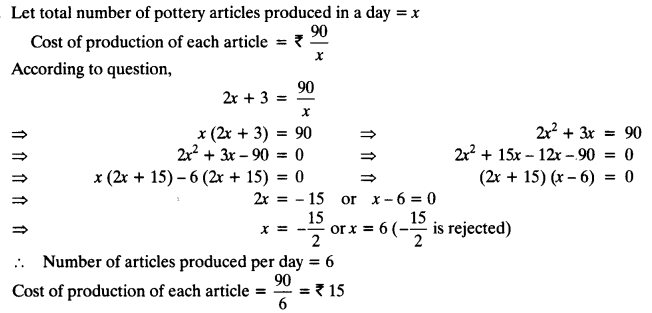We hope the NCERT Solutions for Class 10 Maths Chapter 4 Quadratic Equations Ex 4.2 help you. If you have any query regarding NCERT Solutions for Class 10 Maths Chapter 4 Quadratic Equations Ex 4.2, drop a comment below and we will get back to you at the earliest.